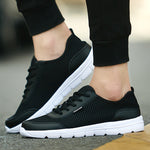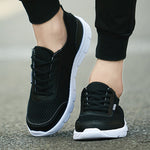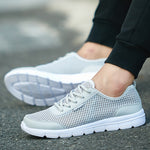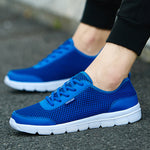Sale
••••# Chris Shoes

- Comfortable Mens Mesh Flats Shoe

- Insole Material: PU
- Outsole Material: EVA
- Lining Material: Mesh
- Upper Material: Mesh (Air mesh)
- Closure Type: Lace-Up

- Feature: Hard-Wearing, Breathable, Massage,
Sweat-Absorbant, Anti-Odor, Light

US Sizes:     Foot Length
3.5    =  36     = 23.5cm
4       =  37     = 24.0cm
5       =  38     = 24.5cm
6       =  39     = 25.0cm
7       =  40     = 25.5cm
8       =  41     = 26.0cm
9       =  42     = 26.5cm
10      =  43     = 27.0cm
11       =  44     = 27.5cm
12      =  45     = 28.0cm
13      =  46     = 28.5cm

• \$39.99
• \$70.99